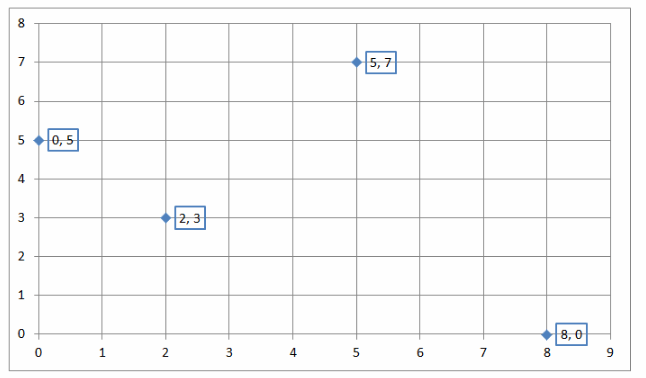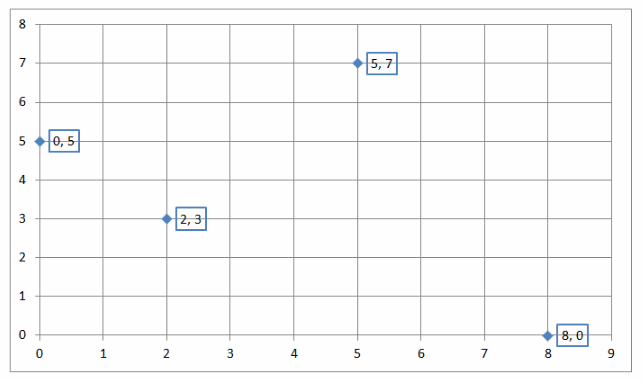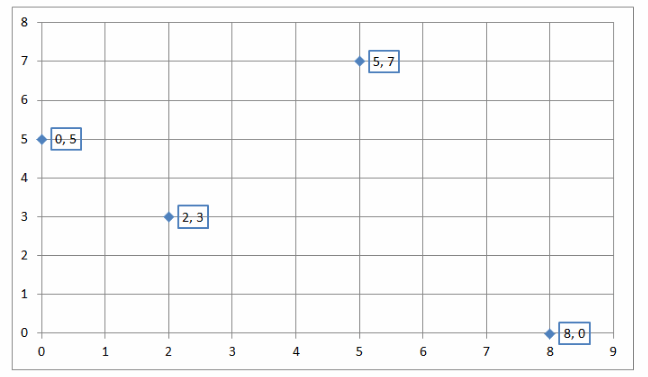# Texas Go Math Grade 8 Module 4 Answer Key Nonproportional Relationships

Refer to our Texas Go Math Grade 8 Answer Key Pdf to score good marks in the exams. Test yourself by practicing the problems from Texas Go Math Grade 8 Module 4 Answer Key Nonproportional Relationships.

## Texas Go Math Grade 8 Module 4 Answer Key Nonproportional Relationships

Essential Question
How can you use non proportional relationships to solve real-world problems?Complete these exercises to review the skills you will need for this chapter.

Find each difference.

Question 1.
3 – (-5) _______
3 – (-5) Given
3 + 5 To subtract an integer, add its opposite.
|3| + |-5| Since the signs are the same, so find the sum of the absolute values
3 + 5 = 8
8 Use the sign of the number with the greater absolute value.

-4 – 5 _______
-4 – 5 Given
-4 + (-5) The signs are the same, so find the sum of the absolute values.
|-4| + |-5|
4 + 5 = 9
-9 Use the sign of the number with the greater absolute value

Question 3.
6 – 10 _____
6 – 10 Given
6 + (-10) The signs are different so find the difference of the absolute values.
(-10) + (6)
|-10| – |6|
10 – 6 = 4
-4 Use the sign of the number with the greater absolute value.

Question 4.
-5 – (-3) ________
-5 – (-3) Given
-5 + 3 To subtract an integer, add its opposite.
|-5| – |3| Since the signs are different, so find the difference of the absolute values
5 – 3 = 2
-2 Use the sign of the number with the greater absolute value

Question 5.
8 – (-8) ________
8 – (-8) Given
8 + 8 To subtract an integer, add its opposite.
|8| + |8| Since the signs are different, so find the difference of the absolute values
8 + 8 = 16
16 Use the sign of the number with the greater absolute value

Module 4 Non-Proportional Relationships Answer Key Question 6.
9 – 5 = _____
9 – 5 Given
9 + (-5) To subtract an integer, add its opposite.
|9| – |5| Since the signs are different, so find the difference of the absolute values
9 – 5 = 4
4 Use the sign of the number with the greater absolute value

Question 7.
-3 – 9 ______
-3 – 9 Given
-3 + (-9) The signs are the same, so find the sum of the absolute values.
|-3| + |-9|
3 + 9 = 12
-12 Use the sign of the number with the greater absolute value
-12

Question 8.
0 – (-6) _____
0 – (-6) Given
0 + 6 To subtract an integer, add its opposite.
|0| + |6| Since the signs are different, so find the difference in the absolute values
0 + 6 = 6
6 Use the sign of the number with the greater absolute value
6

12 – (-9) _____
12 – (-9) Given
12 + 9 To subtract an integer, add its opposite.
|12| + |9| Since the signs are same, so find the difference of the absolute values
12 + 9 = 21
21 Use the sign of the number with the greater absolute value
21

Question 10.
-6 – (-4) _______
-6 – (-4) Given
-6 + 4 To subtract an integer, add its opposite.
|-6| – |4| Since the signs are different, so find the difference of the absolute values
6 – 4 = 2
-2 Use the sign of the number with the greater absolute value

Question 11.
-7 – 10 _______
-7 – 10 Given
-7 + (-10) To subtract an integer, add its opposite.
|-7| + |-10| Since the signs are the same, so find the difference between the absolute values
7 + 10 = 17
-17 Use the sign of the number with the greater absolute value
-17

5 – 14 = ___
5 – 14 Given
5 + (-14) The signs are different, so find the difference of the absolute values
(-14) + (5)
|-14| – |5|
14 – 5 = 11
-11 Use the sign of the number with the greater absolute value
-11

Graph each point on the coordinate grid.Question 13.
B (0, 5)
To graph a point at (0, 5) start at the origin.
Then move 5 points up.
Graph point B(0, 5).To graph a point at (0, 5) start at the origin.
Then move 5 points up.

C (8, 0)Question 15.
D (5, 7)Question 16.
E(2, 3)Visualize Vocabulary
Use the ✓ words to complete the diagram. You can put more than one word in each box.Understand Vocabulary

Complete the sentences using the preview words.

Question 1.
Any ordered pair that satisfies all the equations in a system is a _____